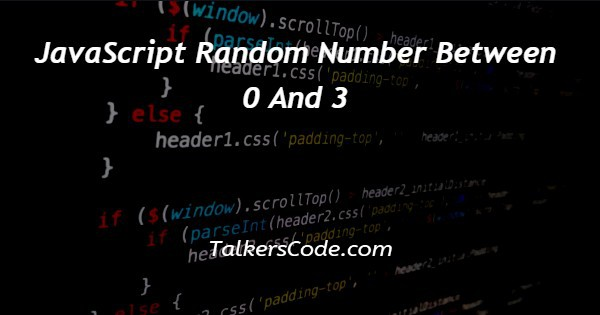# JavaScript Random Number Between 0 And 3

Last Updated : Jan 1, 2023In this tutorial we will show you the solution of JavaScript random number between 0 and 3, today we will learn about how to generate a random number between 0 and 3 with the help of JavaScript.

As, one basic thing to note here that Math.random() function is used here means in JavaScript to generate a random number between 0 and 1. Here, 0 is included but 1 is excluded.

## Step By Step Guide On JavaScript Random Number Between 0 And 3 :-

Math.random() function is only used to generate a random number between 0 and 1. So, we have to modify this method to print number between 0 and 3 which we need.

```<!DOCTYPE html>
<html lang="en">
<meta charset="UTF-8">
<meta name="viewport" content="width=device-width, initial-scale=1.0">
<meta http-equiv="X-UA-Compatible" content="ie=edge">
<body>
<h2>JavaScript Math.random() with Math.floor()</h2>
<button onclick="document.getElementById('demo').innerHTML = getInteger(0,4)">Click Me</button>
<p id="demo"></p>
<script>
function getInteger(min, max) {
return Math.floor(Math.random() * (max - min));
}
</script>
</body>
</html>```
1. First, we write <! DOCTYPE html> which we used as an instruction to the web browser about what version of HTML file is written in.
2. Secondly, the <html> tag is used to indicate the beginning of an HTML document.
3. As above now <head> tag is used to contain information about web page. In this tag a <title> tag is used which helps us to specify a webpage title. Both <head> and <title> tags are Paired tags. So, both have </head> and </title> ending tags respectively.
4. Here, first now body started and body is a paired tag. All the data which we want to show on browser screen is written here.
5. Now, here inside body we create a heading, a button and a paragraph. We create a function on paragraph which work with click on button.
6. Now, inside script we create a function with name getInteger and inside this function we pass two parameters that is min and max. Now, let us go inside function.
7. Inside function we return the value of integer with help of math.random() and math.floor() function. You can see the code for reference.
8. Now, when we click the button. Inside button we use onclick method. With click on button. The value of the demo gets changed and is get from getInteger() function.
9. Inside this function we also pass two parameter that is 0 and 4. Where 0 is the minimum value and 4 is maximum value. It helps us to print a number between 0 and 3 because 4 is excluded.
10. At last, the <body> and <html> tags are closed with </body> and </html> respectively.

## Conclusion :-

At last in conclusion, here we want to say that with the help of this article we are able to print or generate a number between 0 and 3.

I hope this tutorial on JavaScript random number between 0 and 3 helps you and the steps and method mentioned above are easy to follow and implement.